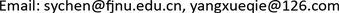1福建师范大学物理与能源学院，福建 福州

2厦门第一中学，福建 厦门1. 引言

2. 惯性系与非惯性系

3. 惯性系在高中力学题目解题的局限性

4. 选用惯性系与非惯性系的解题对比

4.1. 抛体问题

4.2. 追及相遇问题

A. a 1 = a 2 ，A、B相遇一次。 B. a 1 > a 2 ，A、B相遇两次。

C. a 1 < a 2 ，A、B可能不会相遇。 D. a 1 < a 2 ，A、B可能相遇一次。

② a 1 ≠ a 2 时，为关于时间的一元二次方程。由求根公式得 t = − 2 v 0 ± 4 v 0 2 + 8 ( a 1 − a 2 ) L 2 ( a 1 − a 2 ) 。当 a 1 > a 2 ，只有一个正根，即两车相遇一次；当 a 1 < a 2 ，若 Δ < 0 ，方程无解，两车不相遇，若 Δ = 0 ，只有一个正根，即两车相遇一次，若 Δ > 0 ，方程有两解，可能有一个正根，可能有两个正根，因此可能相遇一次，可能相遇两次。

4.3. 滑块木板问题

A先向左做匀减速直线运动至速度为零，后相对于地面向右做匀加速直线运动，加速度大小仍为 a A = 4   m / s 2 。B一直向右做匀减速直线运动。当A相对地面速度为零经历时间 t 1 = 0.5   s ，此时 v B = 1.5   m / s 。A、B再次共速经历时间为 t 2 ，代入公式可解得 t 2 = 0.3   s 。从零时刻到A、B共速过程利用位移公式可以得 L = S A + S B = 0.32   m + 1.28   m = 1.60   m

A相对于B的初速度 v A B = 4   m / s 方向水平向左，A相对于B的加速度 a A B = 5   m / s 。当A、B共速时，A相对于B的速度为零。则利用速度位移公式得 L = 1.60   m 。

4.4. 牵连运动问题

a A B = a B cos θ − a A sin θ 。根据 v A B 2 = a A B l ，可得 a B = a A tan θ + v A 2 l cos 3 θ 。

5. 结语